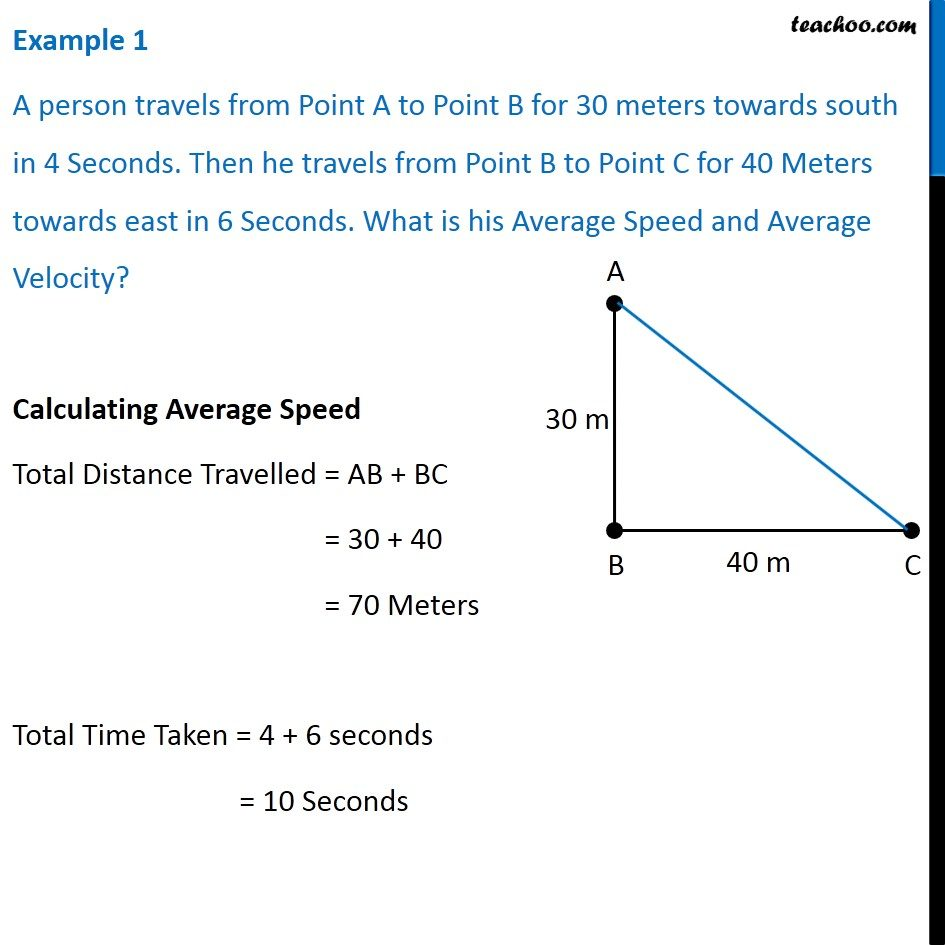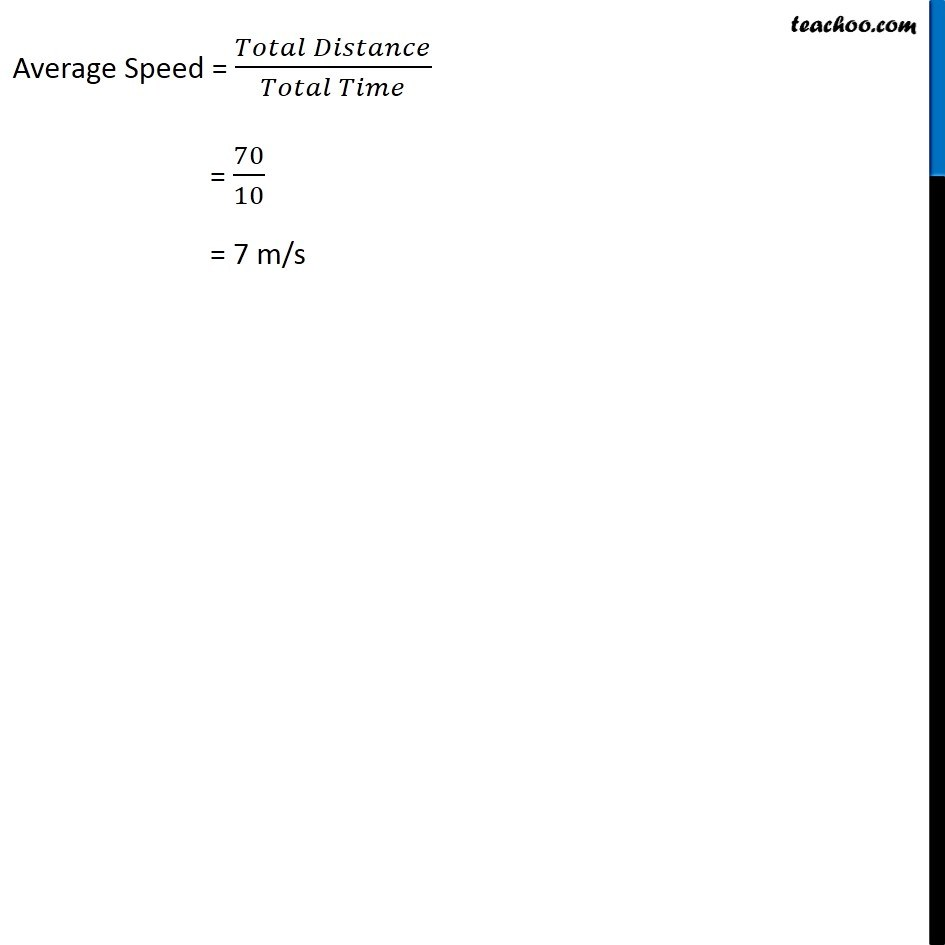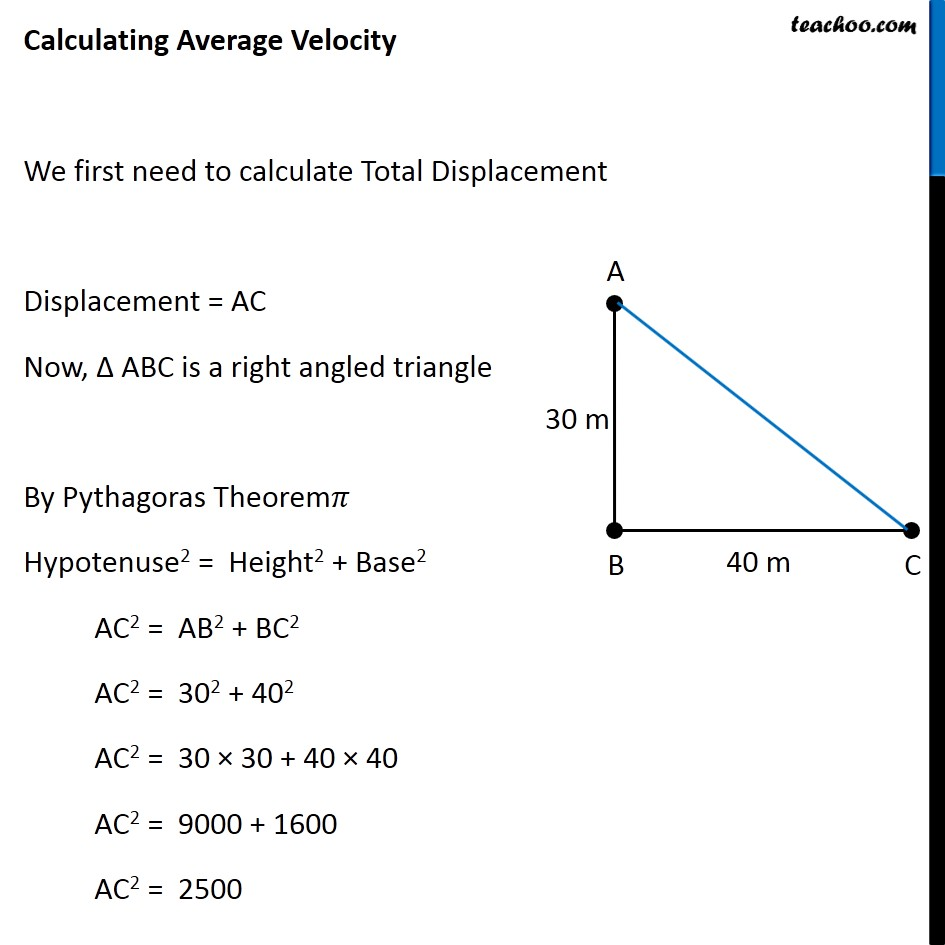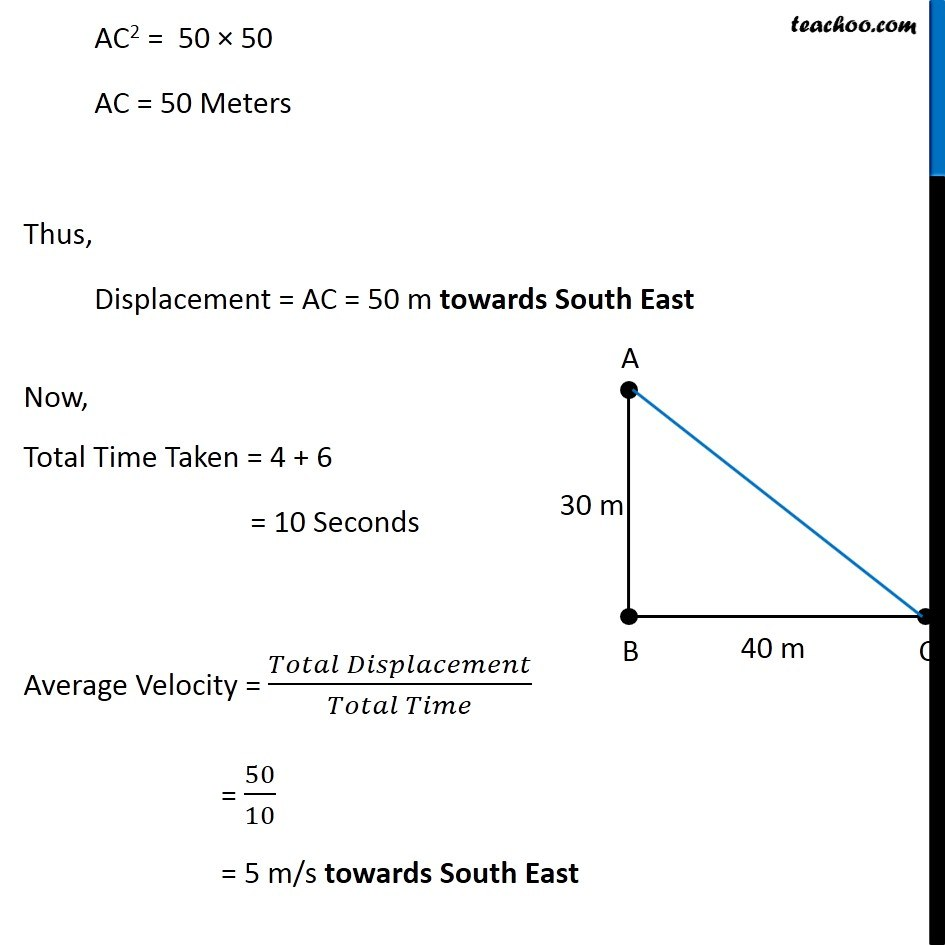Concepts

Class 9
Chapter 8 Class 9 - Motion

## What is Average Velocity?

It is the total distance travelled in a particular diection divided by total time taken

We can say that

Average Velocity = Total Displacement/Total Time Taken

AVERAGE SPEED FORMULA

Average Speed = Total Distance/Total Time Taken

AVERAGE VELOCITY FORMULA

Average Velocity =Total Displacement/Total Time TakenSummary

Average Velocity may or may not be equal to Average Speed

## Questions

Q 2 Page 102 - Under what condition(s) is the magnitude of average velocity of an object equal to its average speed?

NCERT Question 2 - Joseph jogs from one end A to the other end B of a straight 300 m road in 2 minutes 30 seconds and then turns around and jogs 100 m back to point C in another 1 minute. What are Joseph’s average speeds and velocities in jogging (a) from A to B and (b) from A to C?

Example 8.3 - Usha swims in a 90 m long pool. She covers 180 m in one minute by swimming from one end to the other and back along the same straight path. Find the average speed and average Velocity of Usha.

Learn in your speed, with individual attention - Teachoo Maths 1-on-1 Class

### Transcript

Example 1 A person travels from Point A to Point B for 30 meters towards south in 4 Seconds. Then he travels from Point B to Point C for 40 Meters towards east in 6 Seconds. What is his Average Speed and Average Velocity? Calculating Average Speed Total Distance Travelled = AB + BC = 30 + 40 = 70 Meters Total Time Taken = 4 + 6 seconds = 10 Seconds Average Speed = (𝑇𝑜𝑡𝑎𝑙 𝐷𝑖𝑠𝑡𝑎𝑛𝑐𝑒)/(𝑇𝑜𝑡𝑎𝑙 𝑇𝑖𝑚𝑒) = 70/10 = 7 m/s Calculating Average Velocity We first need to calculate Total Displacement Displacement = AC Now, Δ ABC is a right angled triangle By Pythagoras Theorem Hypotenuse2 = Height2 + Base2 AC2 = AB2 + BC2 AC2 = 302 + 402 AC2 = 30 × 30 + 40 × 40 AC2 = 9000 + 1600 AC2 = 2500 AC2 = 50 × 50 AC = 50 Meters Thus, Displacement = AC = 50 m towards South East Now, Total Time Taken = 4 + 6 = 10 Seconds Average Velocity = (𝑇𝑜𝑡𝑎𝑙 𝐷𝑖𝑠𝑝𝑙𝑎𝑐𝑒𝑚𝑒𝑛𝑡)/(𝑇𝑜𝑡𝑎𝑙 𝑇𝑖𝑚𝑒) = 50/10 = 5 m/s towards South East